# WoodWool Tiles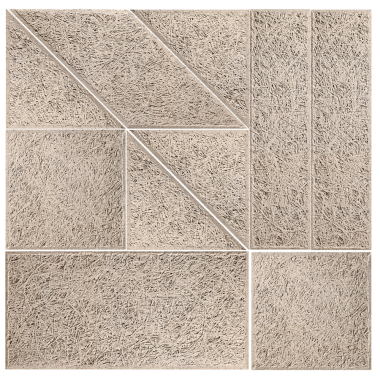Remak® Sound Absorbent Wood Wool Tiles are a recycled, eco-friendly material made from wood fibers, cement and water. These natural ingredients combine to create a product with many outstanding features. Remak® sound absorbing wood bricks are available in 8 shapes; Rectangle, Square, Circle, Hexagon, Parallelogram, Triangle.... with natural thickness from 25; 50 and 75mm.

• Surface:water/natural paint
• Sheet core:Birch fiber + Cement

### Pixel

Area = 0.084m² Equivalent to 11.9 tablets/m²

A = 290 mm

B = 290 mm

C = 10 13 20mm

D = 5mm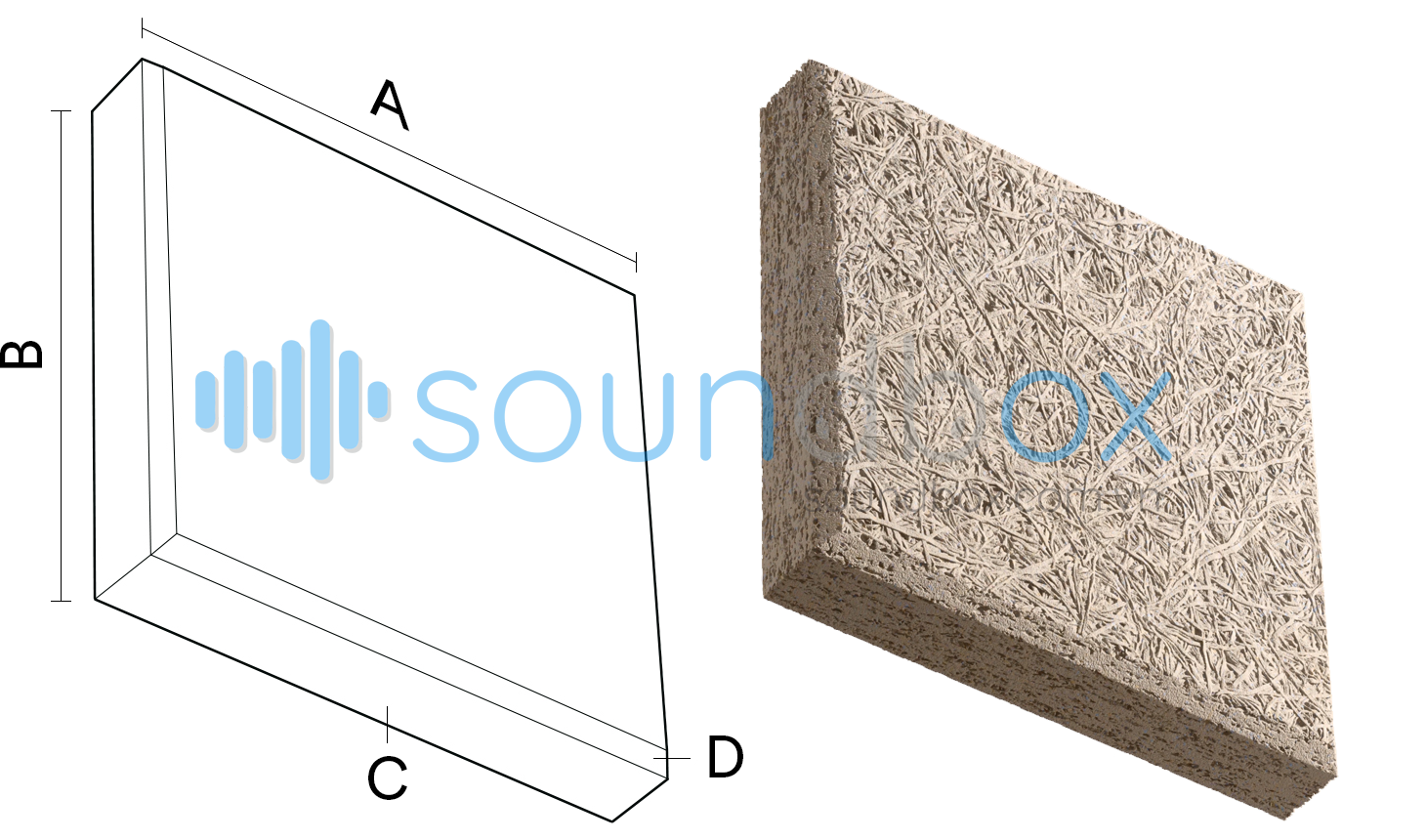### Triangle

Large size: Area = 0.168m² Equivalent to 5.95 tablets/m²

A = 580mm; B = 580mm; C = 5mm

Small size: Area = 0.042m² Equivalent to 23.8 tablets/m²

A = 290 mm; B = 290 mm; C = 5mm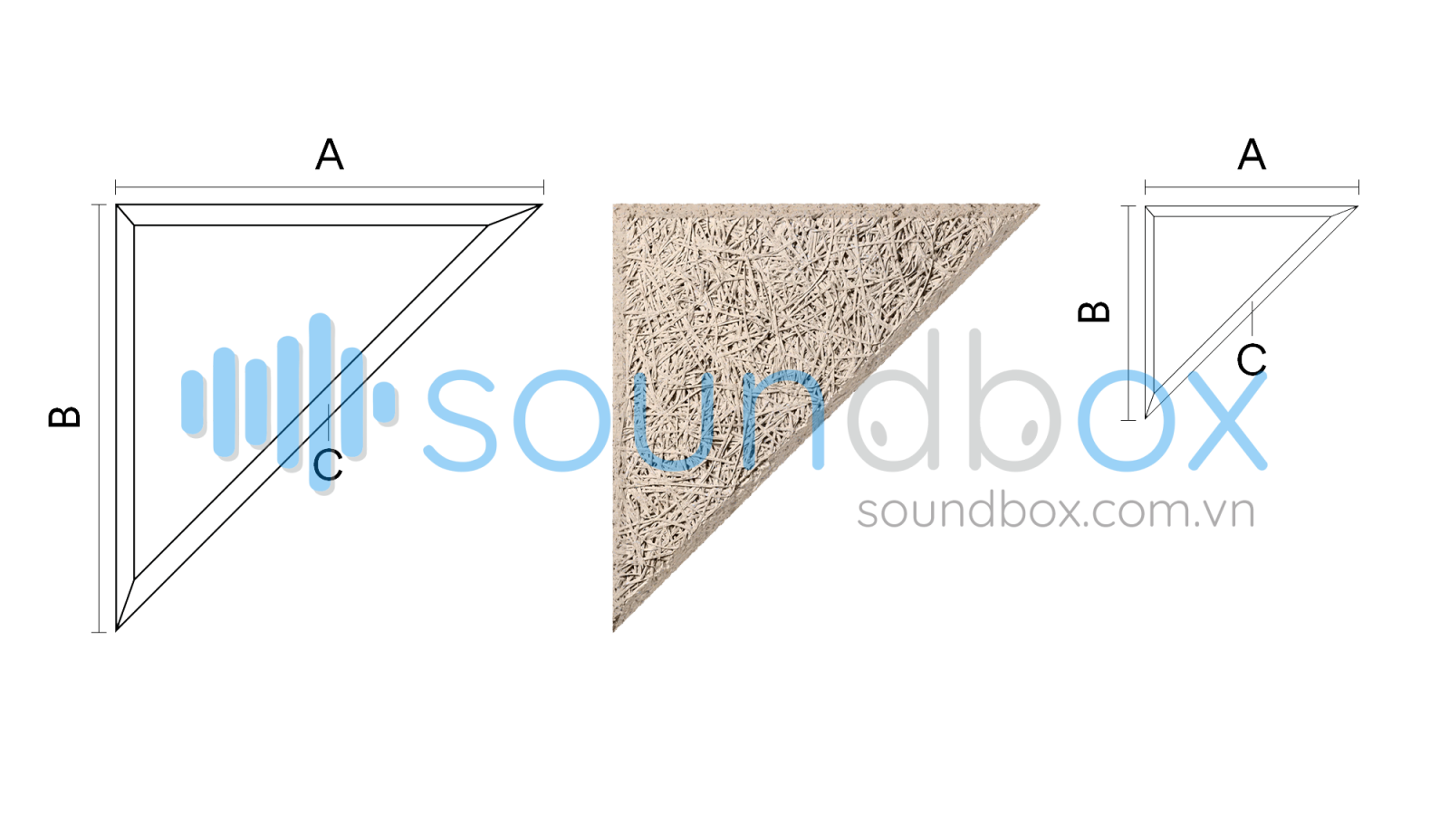### Square

Large size: Area = 0.3364m² Equivalent to 2.97 tablets/m²

A = 580mm; B = 580mm; C = 5mm

Small size: Area = 0.084m² Equivalent to 11.9 tablets/m²

A = 290 mm; B = 290 mm; C = 5mm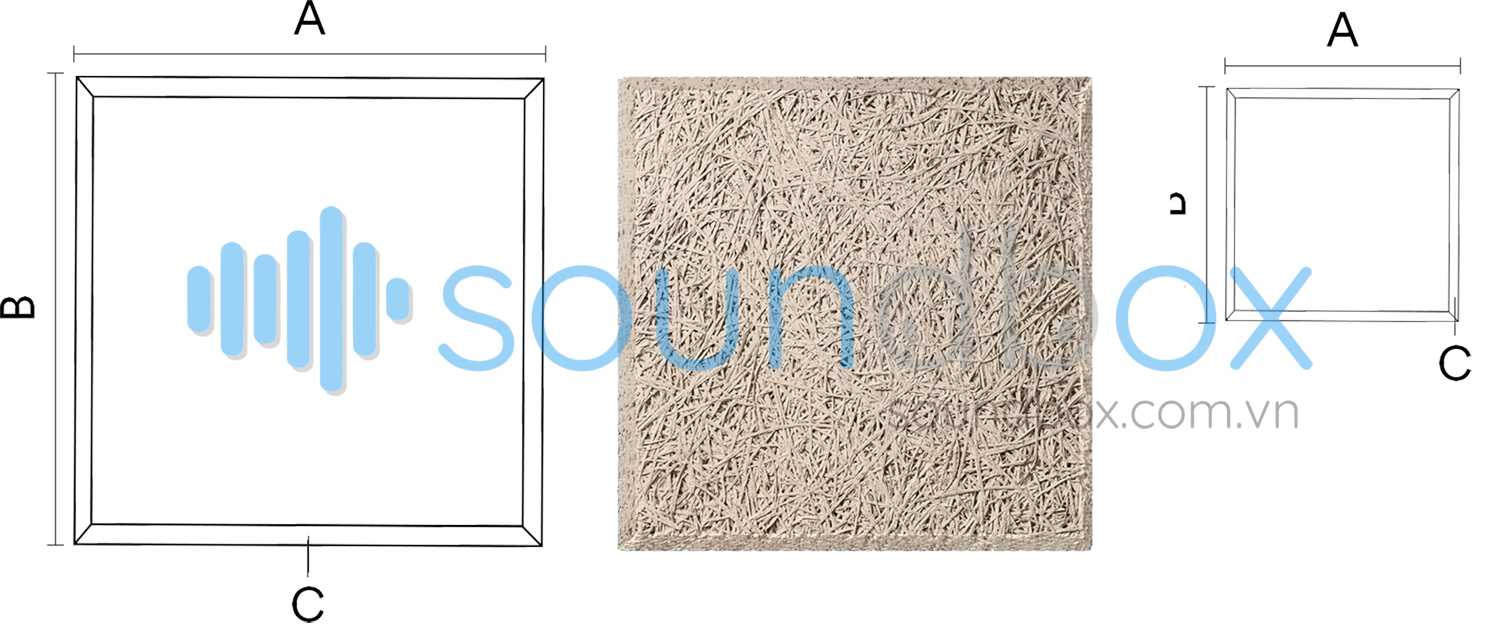### Rectangle

Large size: Area = 0.67m² Equivalent to 1.49 tablets/m²

A = 580mm; B = 1160mm; C = 5mm

Small size: Area = 0.168m² Equivalent to 5.95 tablets/m²

A = 290 mm; B = 580 mm; C = 5mm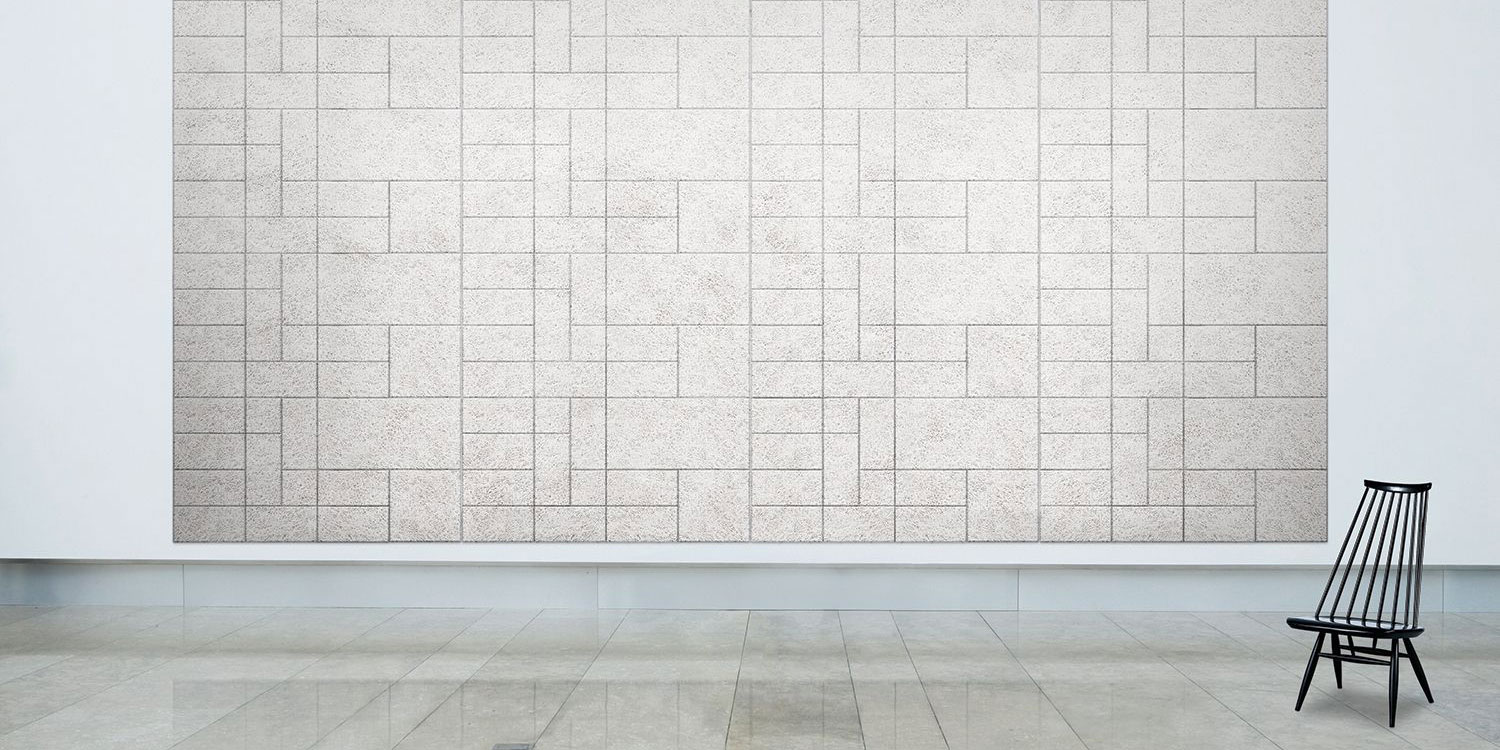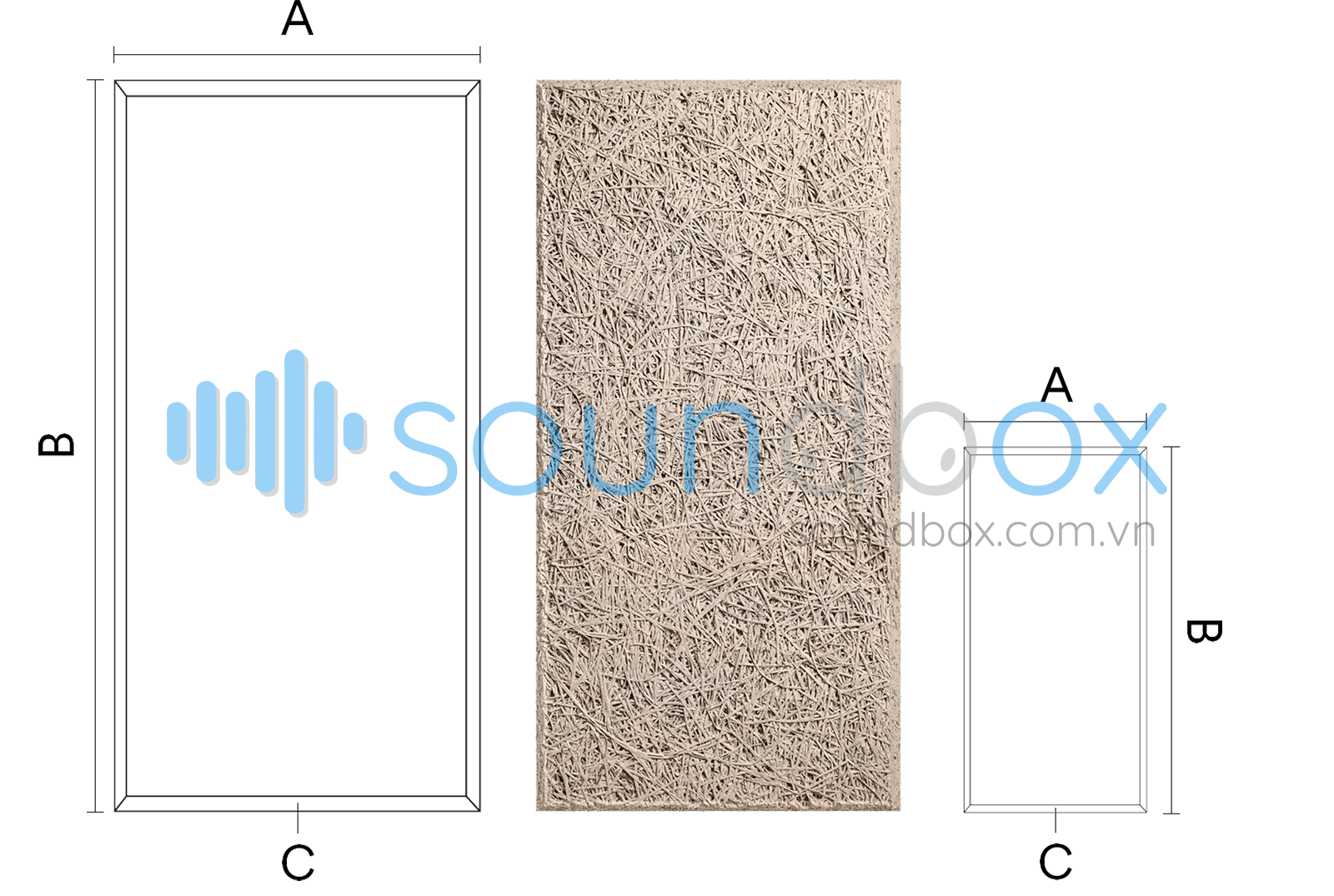### Parallel

Large size: Area = 0.67m² Equivalent to 1.49 tablets/m²

A = 580mm; B = 1160mm; C = 5mm

Small size: Area = 0.084m² Equivalent to 11.9 tablets/m²

A = 290 mm; B = 580 mm; C = 5mm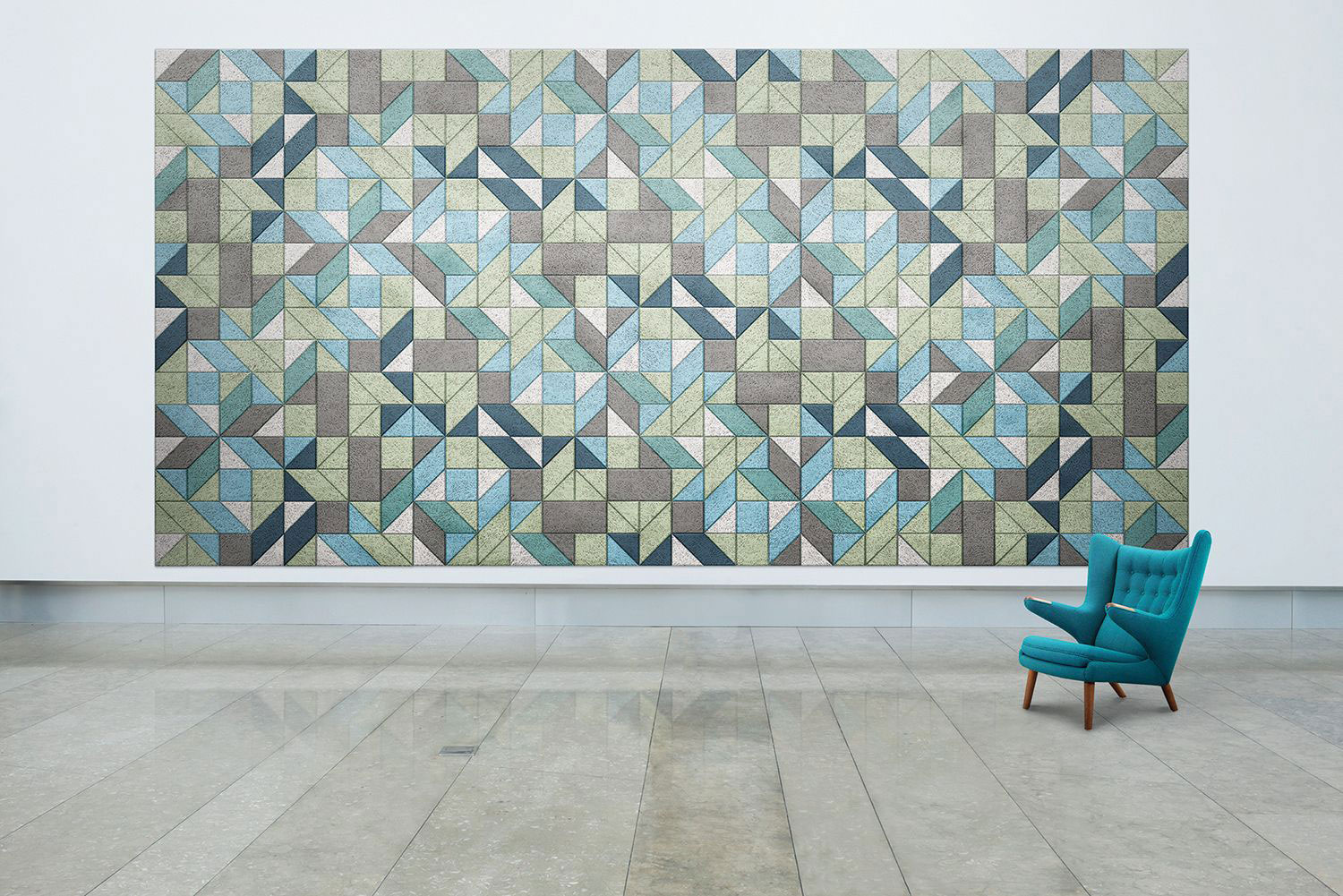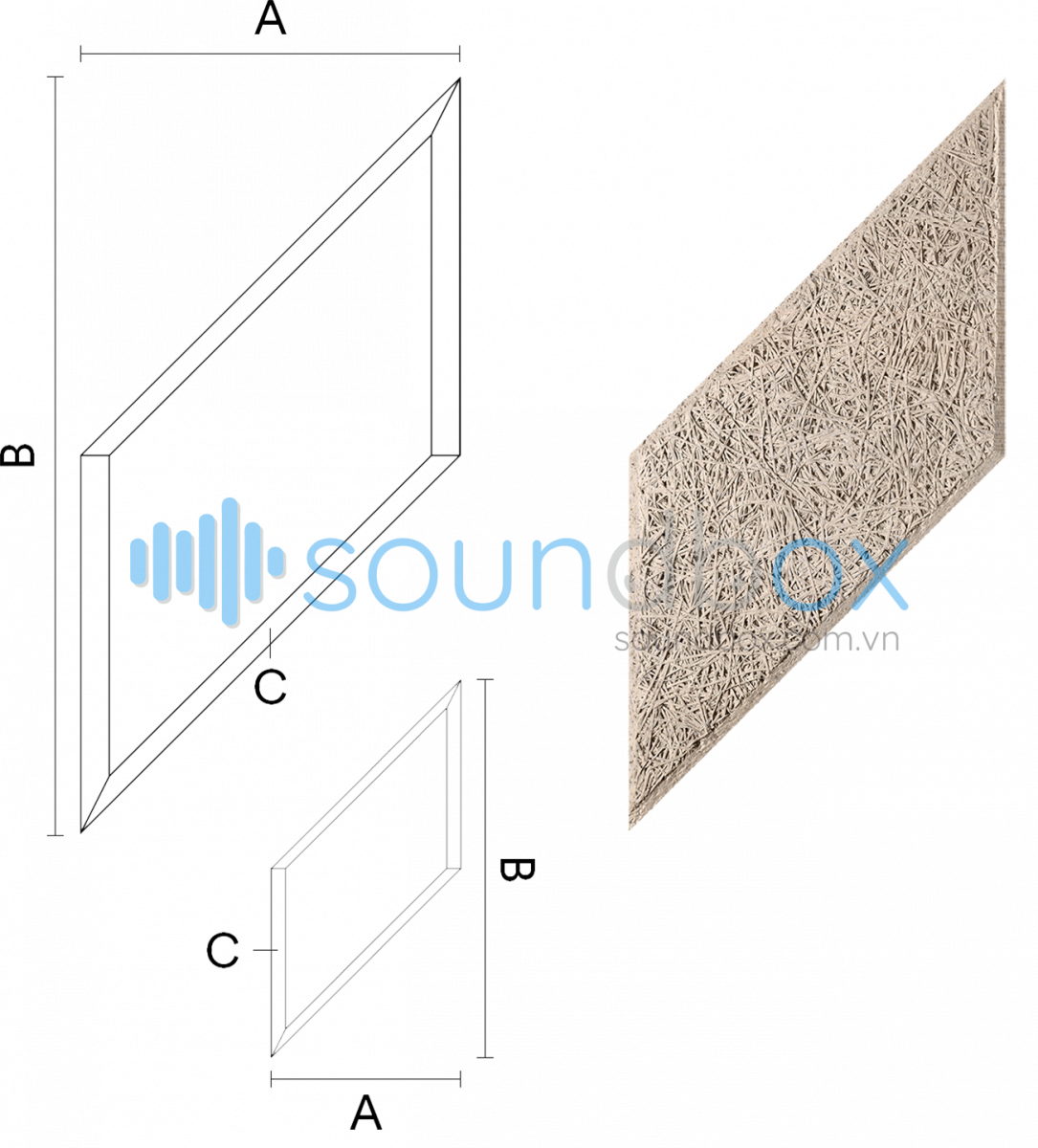### Pank

Large size: Area = 0.3364m² Equivalent to 2.97 tablets/m²

A = 290mm; B = 1160mm; C = 5mm

Small size: Area = 0.084m² Equivalent to 11.9 tablets/m²

A = 145 mm; B = 580 mm; C = 5mm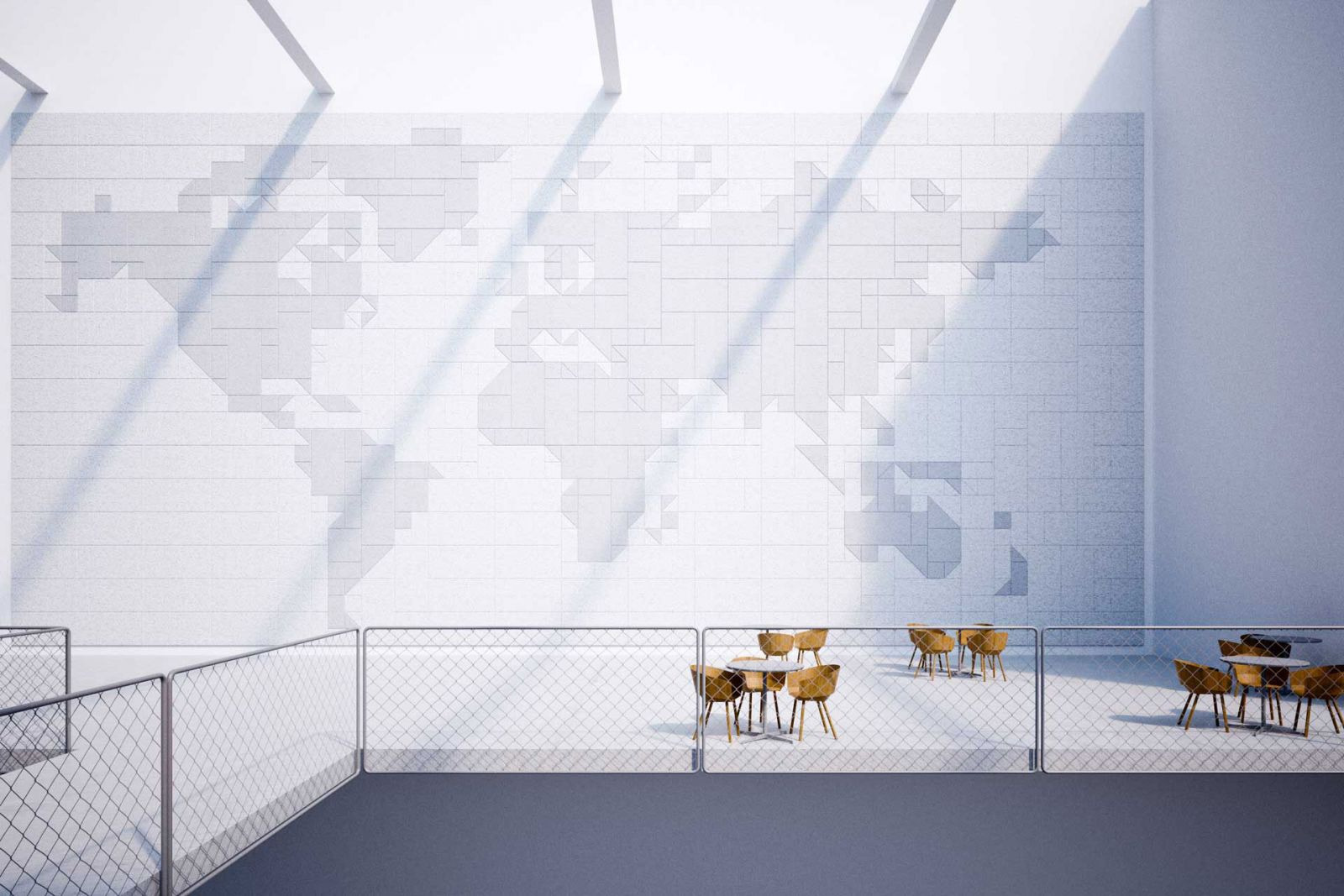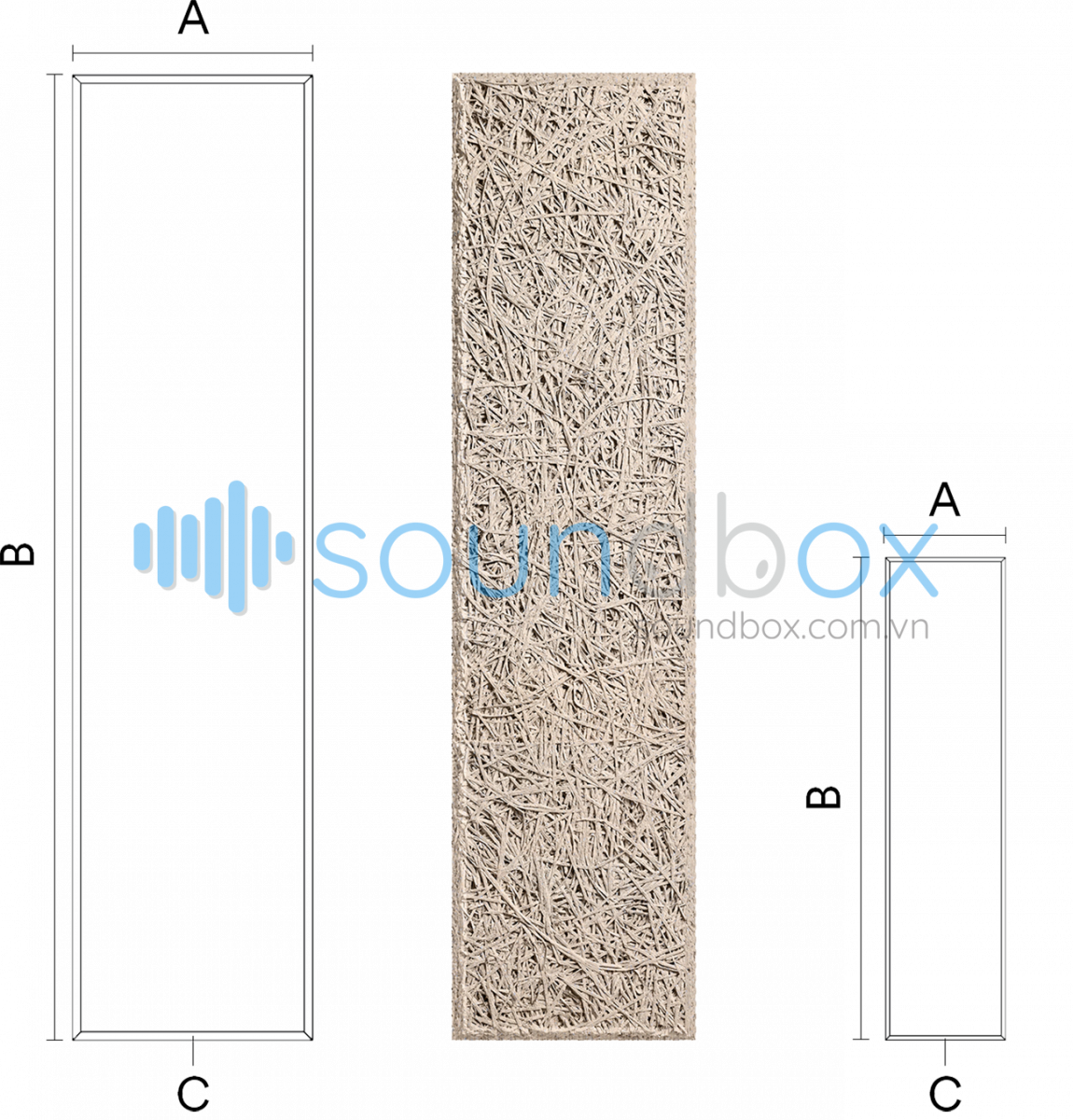### Hexagon

Large size: Area = 0.225m² Equivalent to 4.45 tablets/m²

A = 500mm; B = 580mm; C = 5mm

Small size: Area = 0.056m² Equivalent to 17.8 tablets/m²

A = 250 mm; B = 290 mm; C = 5mm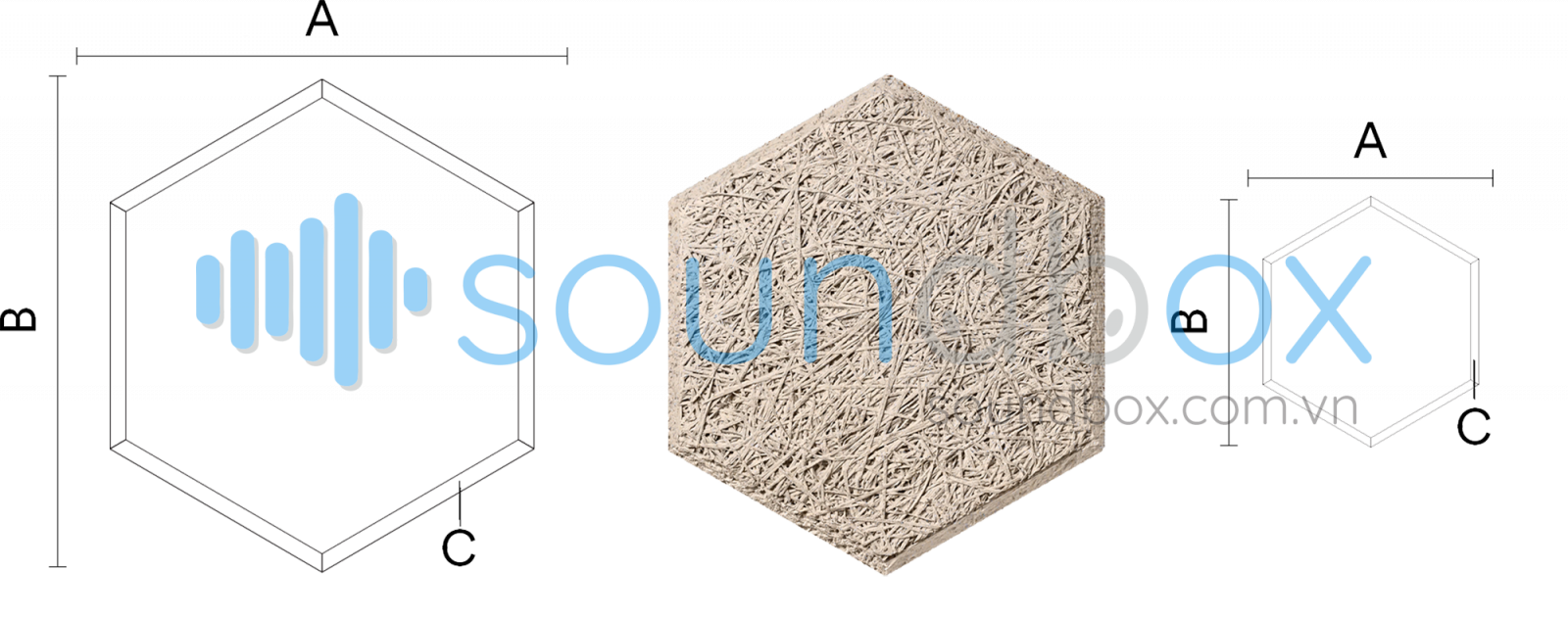### Circle

Large size: Area = 0.264m² Equivalent to 3.78 tablets/m²

A = 580mm; B = 580mm; C = 5 mm

Small size: Area = 0.066m² Equivalent to 15.1 tablets/m²

A = 290 mm; B = 290 mm; C = 5mm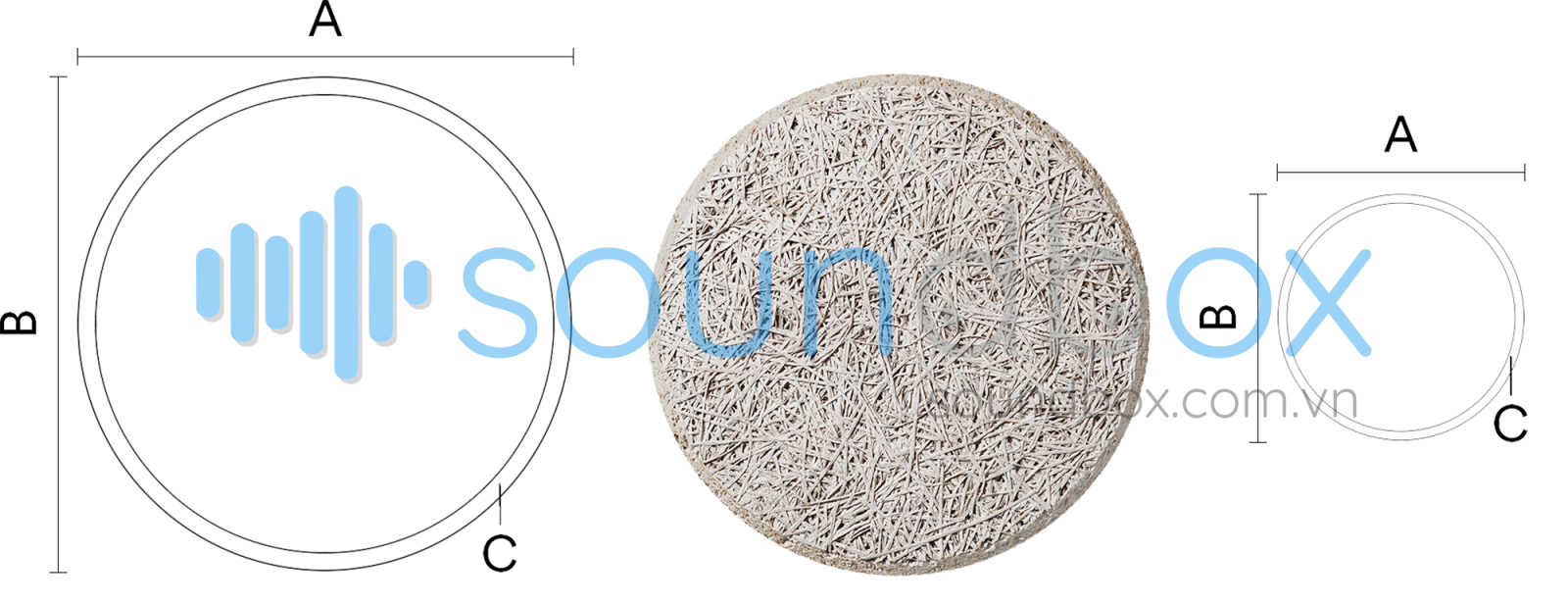### Free to creat with WoodWool Tiles#### Triangle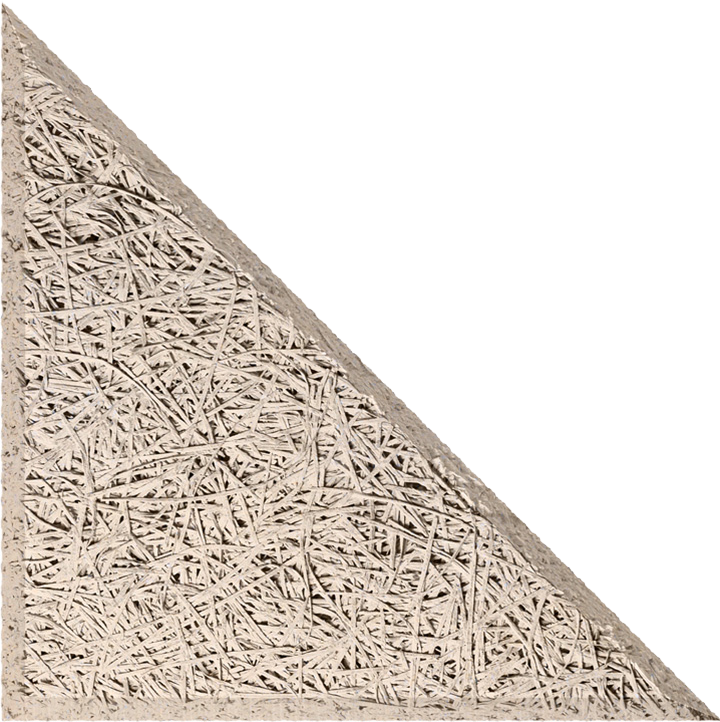#### Square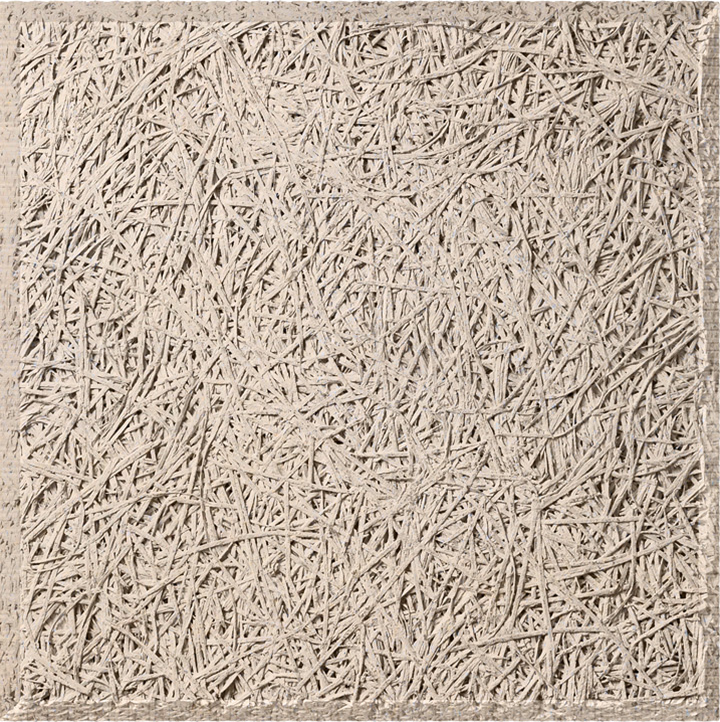#### Rectangle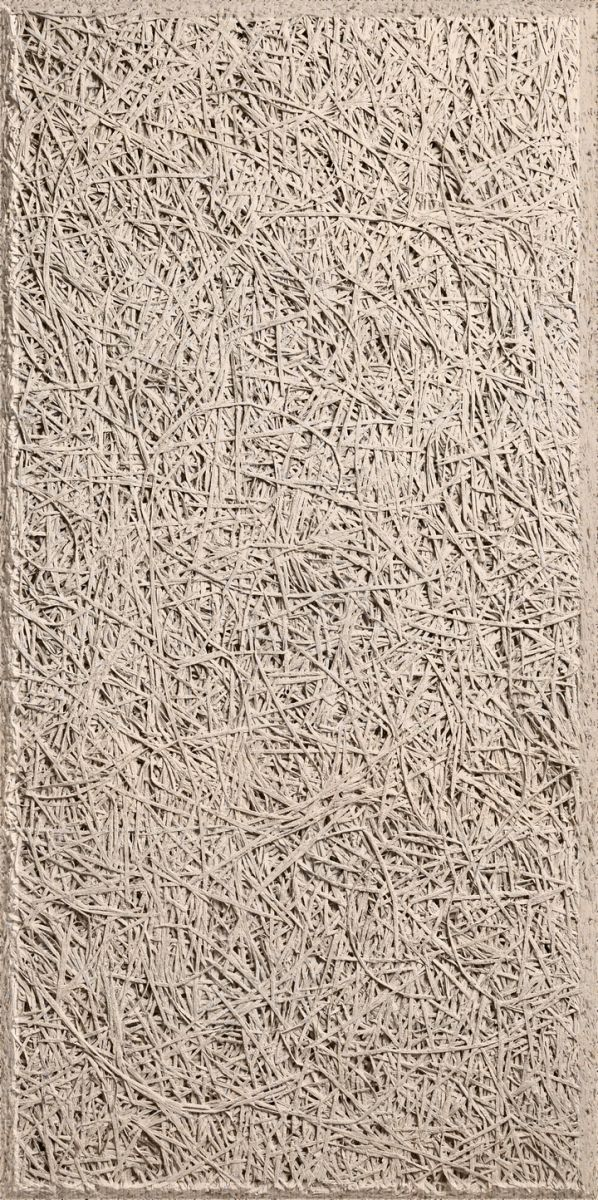​​​​​​

#### Parallel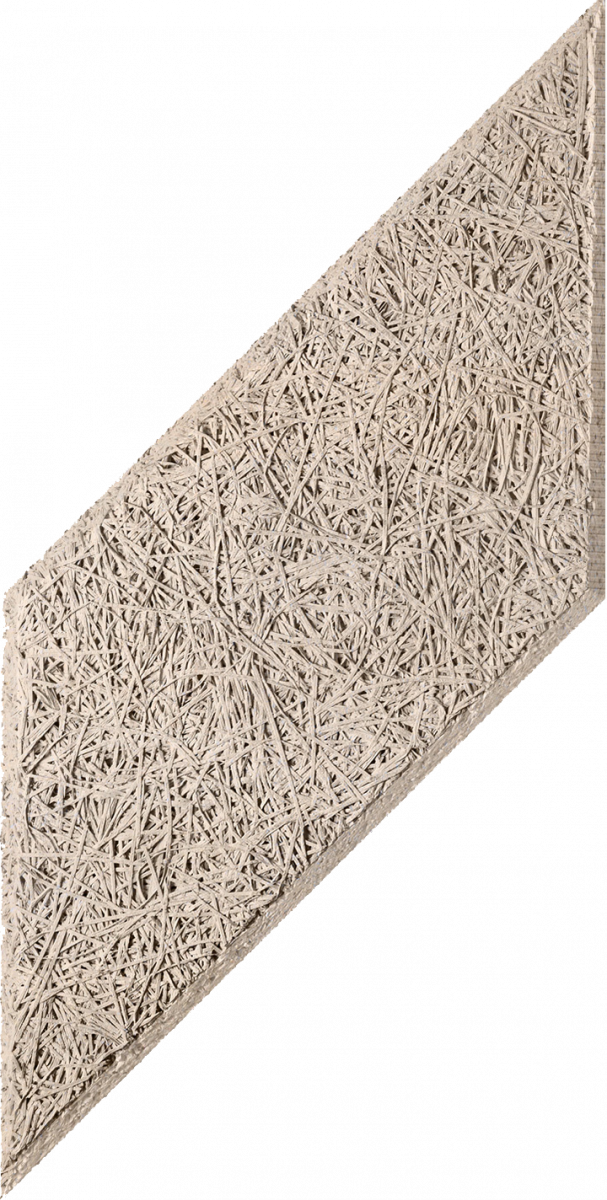​​​​​​

#### Plank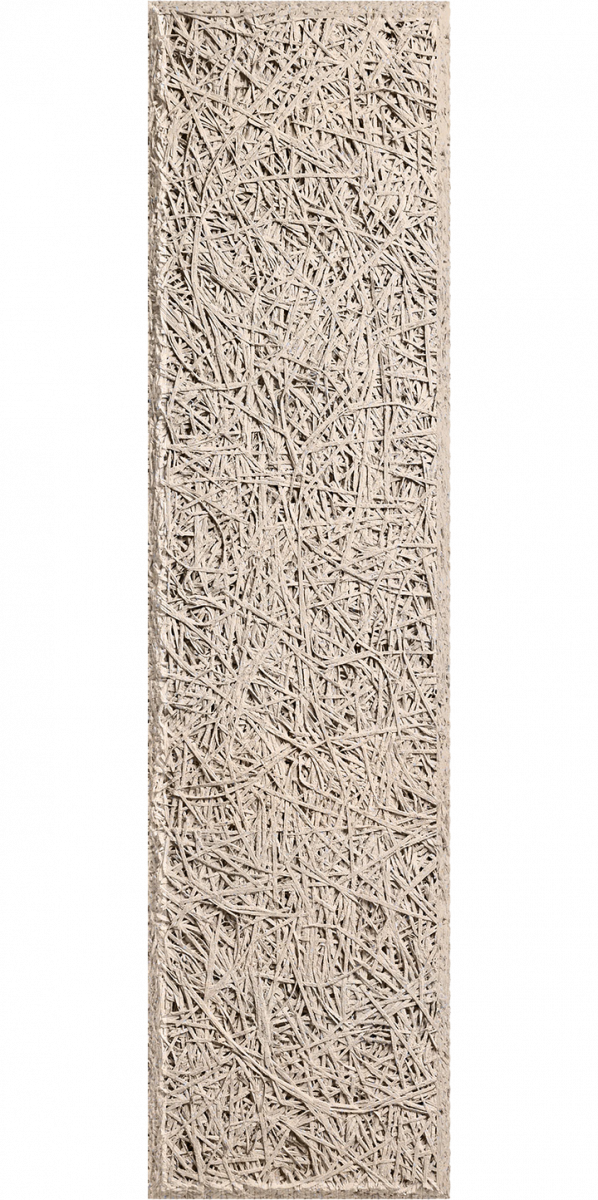#### Hexagon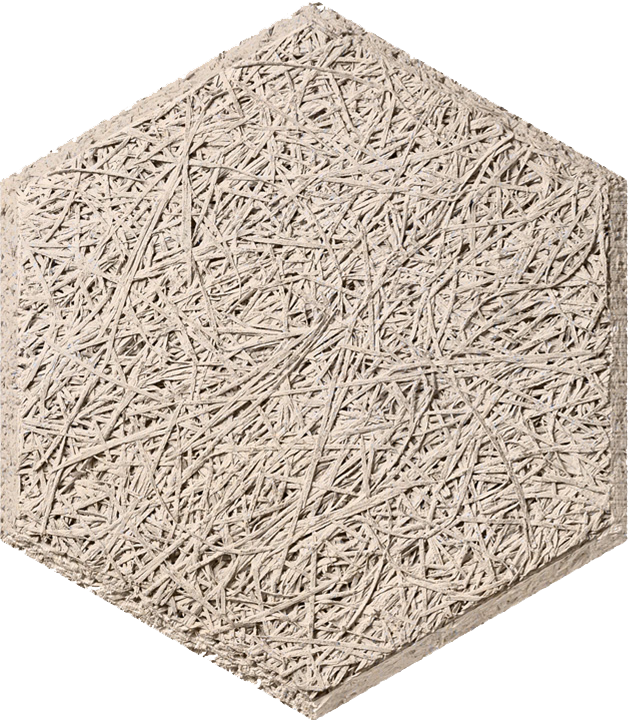​​​​​​

#### Circle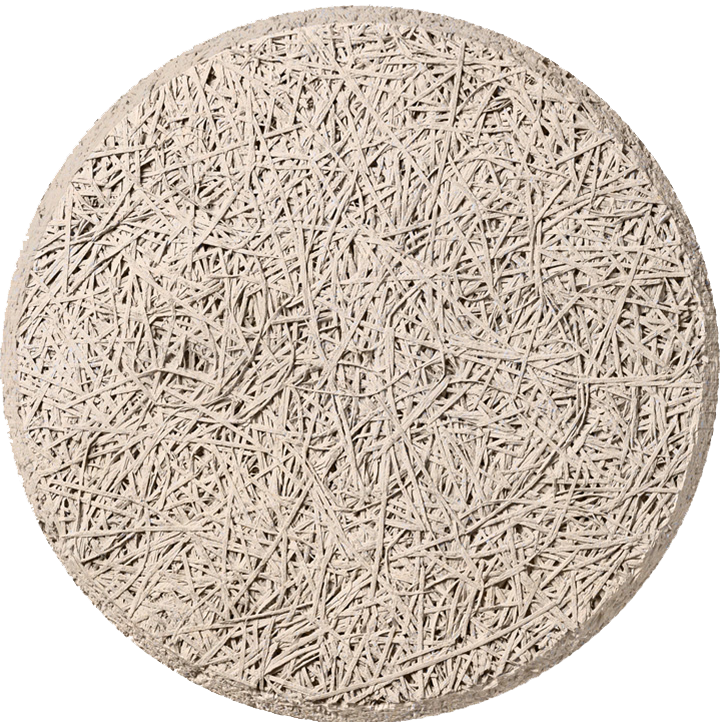GALERY